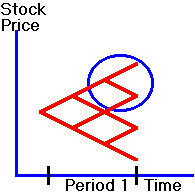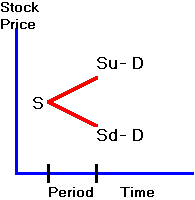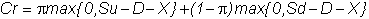﻿ 3.5 Online Pricing Relative to the Yield Curveoffice (412) 967-9367
fax (412) 967-5958
toll-free 1 (800) 214-3480

3.5  Dividends and American Options

You have seen that for an American put option defined on a zero-dividend-paying stock,  the right to early exercise has value, while this is not the case for an equivalent American call option.  Now we take a look at what happens to the call  when the stock pays dividends.

To see that this affects the exercise decision, think of the extreme case where the underlying asset pays out one final liquidating dividend before the option expires.  Clearly, in this case if you have an American call, you would want to exercise early!  Thus there can be value to early exercise if the stock pays dividends.  Of course, if you had a put, you would not exercise.  This already tells you the effect of dividends: they make early exercise of calls more likely and early exercise of puts less likely.

To take a closer look at the exercise value, suppose a dividend will be paid in the period before the call expires.   In Figure 3.5 consider the circled portion of the tree and suppose that a dividend is paid in this period before the call expires.

Figure 3.5

Tree Location Covering the

Dividend Payment PeriodWe will do a one-period analysis from this node. Let the stock price at this node be S, and suppose the stock will pay D per share in the next period, when the call expires.  This means that the price of the stock can be represented as in Figure 3.6.

Figure 3.6

One-Period Model with Dividends(Note that the stock price in the last period must fall by exactly the amount of the dividend; if it falls by more or by less, then there is an arbitrage opportunity.)

If we use risk-neutral valuation, we getNow, substitute the values of the call at expiration to get:If you exercise the call, you receive S - X.  Therefore, if D is large enough relative to Su - X, it will pay you to exercise early.  For example, this is true if Su - D - X = 0 and S - X > 0.

You can repeat this analysis for a put option, where you should find that dividends make early exercise less likely.

You can make these valuation principles more concrete by working through some applications. In  topic 3.6,  Two-Period European versus American Option Example, the two-period analysis is applied to regular European and American options.  In topic 3.7, Two-Period Asian Option Example, we value an "Asian" option.